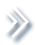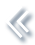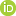####ArchivesReview Articles

## An adaptive lack of fit test for big data

Yanyan Zhao ,

Institute of Statistics and LPMC, Nankai University, Tianjin, ChinaChangliang Zou ,

Institute of Statistics and LPMC, Nankai University, Tianjin, ChinaZhaojun Wang

Institute of Statistics and LPMC, Nankai University, Tianjin, Chinazjwang@nankai.edu.cn

Pages 59-68 | Received 07 Mar. 2017, Accepted 04 Jun. 2017, Published online: 21 Jun. 2017,
• Abstract
• References
• Citations

Abstract

New technological advancements combined with powerful computer hardware and high-speed network make big data available. The massive sample size of big data introduces unique computational challenges on scalability and storage of statistical methods. In this paper, we focus on the lack of fit test of parametric regression models under the framework of big data. We develop a computationally feasible testing approach via integrating the divide-and-conquer algorithm into a powerful nonparametric test statistic. Our theory results show that under mild conditions, the asymptotic null distribution of the proposed test is standard normal. Furthermore, the proposed test benefits from the use of data-driven bandwidth procedure and thus possesses certain adaptive property. Simulation studies show that the proposed method has satisfactory performances, and it is illustrated with an analysis of an airline data.

## References

• Afonja, B. (1972). The moments of the maximum of correlated normal and t-variates. Journal of the Royal Statistical Society B, 34, 251262. [Google Scholar]• Battey, H., Fan, J., Liu, H., Lu, J., & Zhu, Z. (2015). Distributed estimation and inference with statistical guarantees. arXiv:150905457. [Google Scholar]• Chen, X., & Xie, M. (2014). A split-and-conquer approach for analysis of extraordinarily large data. Statistica Sinica, 24, 16551684. [Google Scholar]• Cheng, G., & Shang, Z. (2015). Computational limits of divide-and-conquer method. arXiv:151209226. [Google Scholar]• DasGupta, A. (2008). Asymptotic theory of statistics and probability (1st ed. ). New York, NY: Springer. [Google Scholar]• Eubank, R. L., Ching-Shang, L., & Wang, S. (2005). Testing lack of fit of parametric regression models using nonparametric regression techniques. Statistica Sinica, 15, 135152.• Fan, Y., & Li, Q. (2000). Consistent model specification tests: Kernel-based tests versus Bierens’ ICM tests. Econometric Theory, 16, 10161041. [Google Scholar]• Fan, J., Han, F., & Liu, H. (2014). Challenges of big data analysis. National science review, 1(2), 293314. [Google Scholar]• Gao, J., & Gijbels, I. (2008). Bandwidth selection in nonparametric kernel testing. Journal of the American Statistical Association, 484, 15841594. [Google Scholar]• González-Manteiga, W., & Crujeiras, R. (2013). An updated review of goodness-of-fit tests for regression models. Test, 22, 361411. [Google Scholar]• Guerre, E., & Lavergne, P. (2005). Data-driven rate-optimal specification testing in regression models. Annals of Statistics, 33, 840870. [Google Scholar]• Hall, P. (1984). Central limit theorem for integrated square error of multivariate nonparametric density estimators. Journal of Multivariate Analysis, 14, 116. [Google Scholar]• Hardle, W., & Mammen, E. (1993). Comparing nonparametric versus parametric regression fits. Annals of Statistics, 21, 19261947. [Google Scholar]• Hart, J. (1997). Nonparametric smoothing and lack-of-fit tests (1st ed. ). New York, NY: Springer. [Google Scholar]• Horowitz, J., & Spokoiny, V. (2001). An adaptive, rate-optimal test of parametric mean-regression model against a nonparametric alternative. Econometrica, 69, 599631. [Google Scholar]• Kleiner, A., Talwalkar, A., Sarkar, P., & Jordan, M. I. (2015). A scalable bootstrap for massive data. Journal of the Royal Statistical Society: Series B, 76(4), 795816. [Google Scholar]• Kulasekera, K., & Wang, J. (1997). Smoothing parameter selection for power optimality in testing of regression curves. Journal of the American Statistical Association, 438, 500511. [Google Scholar]• Ledwina, T. (1993). Data-driven version of Neymans smooth test of fit. Journal of the American Statistical Association, 89, 10001005. [Google Scholar]• Lin, N., & Xi, R. (2011). Aggregated estimating equation estimation. Statistics and Its Inference, 4, 7383. [Google Scholar]• Powell, J., Stock, J., & Stoker, T. (1989). Semiparametric estimation of index coefficients. Econometrics, 57, 14031430. [Google Scholar]• Schifano, E., Wu, J., Wang, C., Yan, J., & Chen, M.-H. (2016). Online updating of statistical inference in the big data setting. Technometrics, 58, 393403. [Taylor & Francis Online], [Google Scholar]• Zhang, C. (2003a). Adaptive tests of regression functions via multiscale generalized likelihood ratios. Canadian Journal of Statistics, 31, 151171. [Google Scholar]• Zhang, C. (2003b). Calibrating the degrees of freedom for automatic data smoothing and affective curve checking. Journal of the American Statistical Association, 98, 609629. [Taylor & Francis Online], [Google Scholar]• Zhang, Y., John, D., & Martin, W. (2013). Divide and conquer kernel ridge regression. Journal of Machine Learning Research WCP, 30, 592617. [Google Scholar]• Zhao, T., Cheng, G., & Liu, H. (2016). A partially linear framework for massive heterogeneous data. Annals of Statistics, 44, 14001437. [Google Scholar]• Zheng, J. (1996). A consistent test of functional form via nonparametric estimation techniques. Journal of Econometrics, 75, 263289. [Google Scholar]